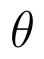Select Page

# CBSE 12 Science Solutions for MCQ Maths Three Dimensional Geometry in English

CBSE 12 Science Solutions for MCQ Maths Three Dimensional Geometry in English to enable students to get Solutions in a narrative video format for the specific question.

Expert Teacher provides CBSE 12 Science Solutions for MCQ Maths Three Dimensional Geometry through Video Solutions in English language. This video solution will be useful for students to understand how to write an answer in exam in order to score more marks. This teacher uses a narrative style for a question from Three Dimensional Geometry not only to explain the proper method of answering question, but deriving right answer too.

Please find the question below and view the Solution in a narrative video format.

Question:

Solution Video in English:

## Similar Questions from CBSE, 12th Science, Maths, Three Dimensional Geometry

Question 1 : A plane makes intercepts -6, 3, 4 respectively on the co-ordinte axes. Find the length of the perpendicular from the origin on it. (View Answer Video)

Question 2 : Find the equation of the plane passing through the line of intersection of the planesandwhich is perpendicular to the plane. (View Answer Video)

Question 3 : If a line marks anglesandwith x, y and z-axis respectively, whereis acute, then find. (View Answer Video)

Question 4 : Find the vector equation of a plane which is at a distance of 5 units from the origin and its normal vector is(View Answer Video)

Question 5 : If linesandintersect, then find the value of k and hence find the equation of plane containing these lines. (View Answer Video)

### Matrices

Question 1 : Find the value of x from the equation:. (View Answer Video)

Question 2 : Find the inverse of the matrix,. (View Answer Video)

Question 3 : Find the transpose of the matrix:. (View Answer Video)

Question 4 : Find the value of z,  from the equation:(View Answer Video)

Question 5 : Find the value of Y, ifand(View Answer Video)

### Linear Programming

Question 1 : The objective function is maximum or minimum, which lies on the boundary of the feasible region. (View Answer Video)

### Relations and Functions

Question 1 :  If f is the greatest integer function and g is the modulus function . Write the value of g o f(-1/3) – f o g ( -1/3 ) . (View Answer Video)

Question 2 : Functionsare defined respectively, by, find(View Answer Video)

Question 3 : Let A ={1, 2, 3}. Then number of equivalence relations containing (1, 2) is: (View Answer Video)

Question 4 : The law a + b = b + a is called ______. (View Answer Video)

Question 5 : A functiondefined as f(x) = 5x is, (View Answer Video)